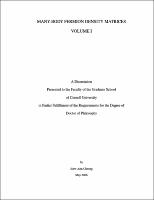## Many-body fermion density matrices##### Abstract
This four-part thesis is on the reduced many-body density matrices of systems of noninteracting and interacting spinless fermions, and the exact solution of ladder models of interacting spinless fermions. In the first part (Chapters 2 and 3), we derived an exact formula relating the density matrix and Green function for a cluster of sites within a system of noninteracting spinless fermions in any dimensions. Based on the thermodynamic form of the cluster density matrix in this exact formula, we proposed a truncation scheme in which the new Hilbert space is built from a truncated set of spinless fermion operators. In the second part (Chapter 4), we studied various finite size effects in the cluster density-matrix spectra, and looked at how these can be reduced or eliminated using the method of twist boundary conditions averaging, for finite two-dimensional systems of noninteracting and interacting spinless fermions. We also checked the feasibility of the operator-based truncation scheme for interacting systems. In the third part (Chapters 5, 6, and 8), we developed a systematic and unbiased machinery, based on the decomposition of the density matrix of two disjoint clusters $a$ and $b$, into a sum of products of an operator on cluster $a$ and an operator on cluster $b$, to extract the various quantum-mechanical correlations, from a numerical exact-diagonalization ground-state wave function. This machinery was applied to explore the ground-state phase diagram of the extended Hubbard ladder of spinless fermions with correlated hops (which are next-nearest-neighbor hops that occur in the presence of occupied nearest neighbors).
##### Description
Ph.D. thesis, submitted January 2006, supervisor Prof. Christopher Henley.